# Regression! Classification! & Clustering!

Regression is a statistical method that can be used in such scenarios where one feature is dependent on the other features. Regression also identifies the importance of the features, the influences of each other, what can be useful, and what can be ignored. Regression usually works well with numerical datasets. Let’s get to the point, I will use the dataset of real estate sales transactions to predict the price-per-unit of a property based on its features. The price-per-unit in this data is based on a unit measurement of 3.3 square meters. The dataset can be found here!

Lets start with loading the dataset and see the features and labels!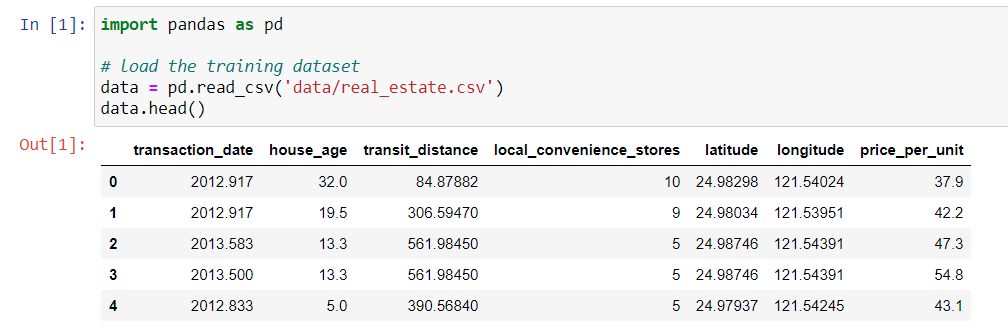Pandas to Load Dataset!

The data consists of the following variables:

transaction_date — the transaction date (for example, 2013.250=2013 March, 2013.500=2013 June, etc.)

house_age — the house age (in years)

transit_distance — the distance to the nearest light rail station (in meters)

local_convenience_stores — the number of convenience stores within walking distance

latitude — the geographic coordinate, latitude

longitude — the geographic coordinate, longitude

price_per_unit house price of unit area (3.3 square meters)

The very important job is to look for missing values and outliers in the dataset. Okay, let’s do that!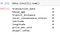No Missing values!

# How about outliers!?

# let’s look into price distribution

import pandas as pd
import matplotlib.pyplot as plt

# This ensures plots are displayed inline in the Jupyter notebook
%matplotlib inline

# Get the label column
label = data[‘price_per_unit’]

# Create a figure for 2 subplots (2 rows, 1 column)
fig, ax = plt.subplots(2, 1, figsize = (9,12))

# Plot the histogram
ax.hist(label, bins=100)
ax.set_ylabel(‘Frequency’)

# Add lines for the mean, median, and mode
ax.axvline(label.mean(), color=’magenta’, linestyle=’dashed’, linewidth=2)
ax.axvline(label.median(), color=’cyan’, linestyle=’dashed’, linewidth=2)

# Plot the boxplot
ax.boxplot(label, vert=False)
ax.set_xlabel(‘Prices’)

# Add a title to the Figure
fig.suptitle(‘Price Distribution’)

# Show the figure
fig.show()

The output will be: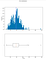Outliers Exist in Price_per_units!

We can remove the outliers by trimming the dataset. In the above figure, we can see that the highest value on the right side around 70. After 70, those values are considered as outliers. So removing the outliers will look like this:

# Remove Outliers
data = data[data[‘price_per_unit’] < 70]

So, if we visualize it again, it will look like this: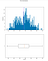Outliers Removed!

Now, I think we did enough with the data to apply regression. However, it is always good practice to normalize the data to keep them in the same space.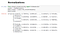Normalizing the Data!

After normalizing the data, we need to separate features and labels. We also need to use train_test_split to split the data into training and testing sets.

# Separate features and labels
X, y = data[[‘transaction_date’, ‘house_age’, ‘transit_distance’, ‘local_convenience_stores’, ‘latitude’, ‘longitude’]].values, data[‘price_per_unit’].values

# Split data 70%-30% into training set and test set

from sklearn.model_selection import train_test_split

X_train, X_test, y_train, y_test = train_test_split(X, y, test_size=0.30, random_state=0)

We split the dataset into 70% training and 30% testing data. Now, it is time to apply regression algorithms and fit the model with the data.

# Train the model
from sklearn.linear_model import LinearRegression

# Fit a linear regression model on the training set
model = LinearRegression().fit(X_train, y_train)

predictions = model.predict(X_test)

In this case, we have used the Linear Regression algorithm to fit the model and make the predictions using the testing data. Okay, let’s visualize our regression model based on label test and prediction data.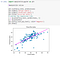Pretty good regression model!

We can also use Mean Squared Error (MSE) and R2 Score to evaluate the model. MSE actually identifies how close a regression line is to a set of points. The equation for MSE is given below: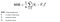Taken From WikiPedia!

“R-squared (R2) is a statistical measure that represents the proportion of the variance for a dependent variable that’s explained by an independent variable or variables in a regression model”. A detail descriptions can be found here!

from sklearn.metrics import mean_squared_error, r2_score
from sklearn.ensemble import GradientBoostingRegressor

# Fit a lasso model on the training set
model = GradientBoostingRegressor().fit(X_train, y_train)
print (model, “\n”)

# Evaluate the model using the test data
predictions = model.predict(X_test)
mse = mean_squared_error(y_test, predictions)
print(“MSE:”, mse)
rmse = np.sqrt(mse)
print(“RMSE:”, rmse)
r2 = r2_score(y_test, predictions)
print(“R2:”, r2)

The output will be like the following: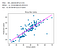MSE and R2!

Okay, enough about regression. Let’s talk about classification now!

Classification usually falls into the category of recognizing something based on a pre-existing training dataset. In the classification problem, each features combined to make a label. Therefore, there can be multiple labels exists in a dataset. For example, recognizing the fingers from the hand would be a classification problem. In this case, I will be using the Wine Classification problem. The dataset can be found here! Let’s start by loading the dataset: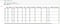Wine Classification Dataset!

And the labels are:

• 0 (variety A)
• 1 (variety B)
• 2 (variety C)

Now, follow the same steps to clean the data and remove the outliers as we have seen in the regression part. Therefore, we have to separate the dataset into a training and testing set.

features = [‘Alcohol’, ‘Malic_acid’, ‘Ash’, ‘Alcalinity’, ‘Magnesium’, ‘Phenols’,
‘Flavanoids’, ‘Nonflavanoids’, ‘Proanthocyanins’, ‘Color_intensity’,
‘Hue’, ‘OD280_315_of_diluted_wines’, ‘Proline’]
label = ‘WineVariety’
X, y = data[features].values, data[label].values

from sklearn.model_selection import train_test_split
X_train, X_test, y_train, y_test = train_test_split(X, y, test_size = 0.30, random_state=0)
print(‘Training Class: %d\nTestig Class: %d’ % (X_train.shape, X_test.shape))

And the output would be:

`Training Class: 124Testig Class: 54`

From the above code, it is clear that we have taken 70% of the data as a training set and 30% of the data as a testing set.

Now, I am going to use sklearn pipelining to preprocess the dataset and fed them into a classifier. Pipeline is just an abstract notion, it’s not some existing ml algorithm. Often in ML tasks, you need to perform a sequence of different transformations (find set of features, generate new features, select only some good features) of the raw dataset before applying final estimator.” Also, we can look into the details of pipelining here!

from sklearn.preprocessing import StandardScaler
from sklearn.compose import ColumnTransformer
from sklearn.pipeline import Pipeline
from sklearn.linear_model import LogisticRegression

feature_columns = [0,1,2,3,4,5,6]
feature_transformer = Pipeline(steps=[(‘scaler’, StandardScaler())])

# Create preprocessing steps
preprocessor = ColumnTransformer(transformers=[(‘preprocess’, feature_transformer, feature_columns)])

# Create training pipeline
pipeline = Pipeline(steps=[(‘preprocessor’, preprocessor),
(‘regressor’, LogisticRegression(solver=’lbfgs’, multi_class=’auto’))])

# fit the pipeline to train a linear regression model on the training set
model = pipeline.fit(X_train, y_train)

And if we predict the model with the test data, then the model outcome would be: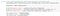Model Evaluations!

Now, I will show how to evaluate the effectiveness of the model by using a confusion matrix. Confusion Matrix is a performance measurement for a machine learning classification problem where output can be two or more classes. It is a table with 4 different combinations of predicted and actual values. It is useful for measuring Recall, Precision, Accuracy, and AUC-ROC curves.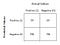Confusion Matrix Combinations!

In the above figure, TP is true positive which means that your prediction is positive and it is true. FP is false positive which means that your prediction is positive but it’s false. FN is false negative which means that your prediction is negative and it’s false. Lastly, TN is true negative which means that your prediction is negative and it’s true. Also, we can calculate the Recall = TP/(TP+FN), precision = TP/(TP+FP), and F-measures = (2*recall*precision)/ (recall + precision). Okay, let’s look at the confusion matrix for our classification problem.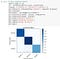Confusion Matrix!

In addition to the confusion matrix, the roc curve also shows how good your classifier is!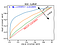Taken from Web!

In the above figure, we can clearly understand that what kind of graph will tell us if the classifier is good or not! Well, let’s look at our ROC curve!

from sklearn.metrics import roc_curve, roc_auc_score

# Get class probability scores
probabilities = model.predict_proba(X_test)

auc = roc_auc_score(y_test,probabilities, multi_class=’ovr’)
print(‘Average AUC:’, auc)

# Get ROC metrics for each class
fpr = {}
tpr = {}
thresh ={}
for i in range(len(classes)):
fpr[i], tpr[i], thresh[i] = roc_curve(y_test, probabilities[:,i], pos_label=i)

# Plot the ROC chart
plt.plot(fpr, tpr, linestyle=’ — ‘,color=’orange’, label=classes + ‘ vs Rest’)
plt.plot(fpr, tpr, linestyle=’ — ‘,color=’green’, label=classes + ‘ vs Rest’)
plt.plot(fpr, tpr, linestyle=’ — ‘,color=’blue’, label=classes + ‘ vs Rest’)
plt.title(‘Multiclass ROC curve’)
plt.xlabel(‘False Positive Rate’)
plt.ylabel(‘True Positive rate’)
plt.legend(loc=’best’)
plt.show()

The output would be: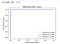ROC curve of our classification!

Therefore, this classification model is the perfect classifier!

Clustering comes in when we can not do the regression and classification of the data. That being said, clustering is distributing the data to its closest group. Clustering is an unsupervised machine learning technique in which you train a model to group similar entities into clusters based on their features.

In this example, I will be using a pre-defined dataset! You can use any dataset you would like! Let’s load the data first!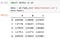Dataset!

The challenge is to identify the number of discrete clusters present in the data and create a clustering model that separates the data into that number of clusters. Another challenge is also to visualize the clusters to evaluate the level of separation achieved by your model. First off, let’s use PCA to make 2D version visualization. “PCA is a dimensionality reduction method that is often used to reduce the dimensionality of large data sets, by transforming a large set of variables into a smaller one that still contains most of the information in the large set.” Therefore, we will be using it to make the dataset into a 2D version and then visualize it.

from sklearn.preprocessing import MinMaxScaler
from sklearn.decomposition import PCA
import matplotlib.pyplot as plt
%matplotlib inline

scaled_features = MinMaxScaler().fit_transform(data)

pca = PCA(n_components=2).fit(scaled_features)
features2D = pca.transform(scaled_features)

plt.scatter(features2D[:,0], features2D[:,1])
plt.xlabel(‘Dimention 1’)
plt.ylabel(‘Dimention 2’)
plt.title(‘Data’)
plt.show()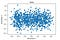2D visualization of the data using PCA!

We can also run multiple cluster size examples using the KMeans clustering algorithm to find the suitable cluster size. Okay, let’s do that!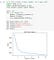Finding suitable cluster size!

In the above figure, we can see that there are four edges or elbows if you call it. These elbows tell us that there can be four possible clusters that exists in this dataset! Cool, right? 😆

Now, we are going to use the KMeans cluster with 4 cluster sizes!

from sklearn.cluster import KMeans

model = KMeans(n_clusters=4, init=’k-means++’, n_init=500, max_iter=1500)
km_clusters = model.fit_predict(data)

In the above code, n_init means 500 times of training and max_iter 1500 means that in each training, there can be a maximum of 1500 iterations. Now, let’s visualize the clusters!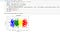Four Clusters!

We have got our clusters right? Yeah looks cool! Clustering can be useful to make the classification as well. Think about it! 😉

That’s it for today! Cheers! 😃

Data Scientists / Full Stack Deep Learning

## More from Mahedi Hasan Jisan

Data Scientists / Full Stack Deep Learning